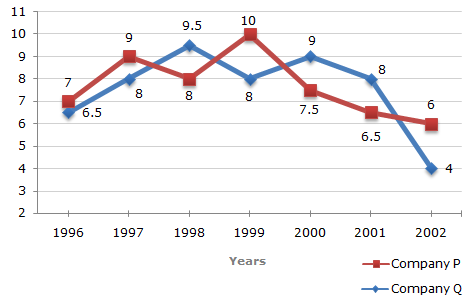# Data Interpretation - Line Charts - Discussion

### Discussion :: Line Charts - Line Chart 8 (Q.No.4)

Two different finance companies declare fixed annual rate of interest on the amounts invested with them by investors. The rate of interest offered by these companies may differ from year to year depending on the variation in the economy of the country and the banks rate of interest. The annual rate of interest offered by the two Companies P and Q over the years are shown by the line graph provided below.

Annual Rate of Interest Offered by Two Finance Companies Over the Years.4.

An investor invested a sum of Rs. 12 lakhs in Company P in 1998. The total amount received after one year was re-invested in the same Company for one more year. The total appreciation received by the investor on his investment was?

 [A]. Rs. 2,96,200 [B]. Rs. 2,42,200 [C]. Rs. 2,25,600 [D]. Rs. 2,16,000

Explanation:

Amount received from Company P after one year (i.e., in 199) on investing Rs. 12 lakhs in it

= Rs. [12 + (8% of 12)] lakhs

= Rs. 12.96 lakhs.

Amount received from Company P after one year on investing Rs. 12.96 lakhs in the year 1999

= Rs. [12.96 + (10% of 12.96)] lakhs

= Rs. 14.256.

Appreciation received on investment during the period of two years

= Rs. (14.256 - 12) lakhs

= Rs. 2.256 lakhs

= Rs. 2,25,600.

 Sourb said: (Oct 8, 2011) How is this possible. Why 8% in first step turned into 10% in next step? Can you please elaborate it.

 Akhila said: (May 3, 2012) In the 1st step, we consider the interest rate of year 1998 and in the next step we consider the interest rate of the next year i.e.10%

 Chip said: (Oct 1, 2012) I got this wrong the first time around because I did not add the interest from the first year to the principal for the second year.

 Annie said: (Jun 18, 2015) I did not understand the last step i.e appreciation received on investment during the period of two years: = Rs. (14.256 - 12) lakhs please explain.

 Pratik said: (Oct 14, 2016) Initial = 1200000, interest in year 1998 = 0.08, So interest amount received = 96000 (= 1200000 * 0.08) . Net amount after investment = 1296000 (=1200000 + 96000) . Next year 1999, interest rate = 0.1, Amount invested = 1296000, Interest received = 1296000 * 0.1= 129600. Total interest (appreciation) = interest in (1998 + 1999) = 96000 + 129600 = 225600 => Answer.

 Ravisha said: (Dec 3, 2016) Thank you all for the explanation.

 Hemjit said: (Jan 17, 2017) An easier way: Net interest rate: 8+10+(8*10/100) = 18.8% -- this would be the combined interest for two years. Interest or appreciation: 12 * 18.8/100 = 225.6/100 = 2.256.

 Sheena said: (Jan 19, 2017) Thanks for the explanation @Pratik.

 Yagnas said: (Jul 7, 2018) Instead of appreciation, here interest would be the correct word.

 Moin Khan Mondal said: (Jun 1, 2019) Total amount =p(1+R1/100)(1+R2/100)=1200000(1+8/100)(1+10/100)=1425600. The answer is = 1425600-1200000 = 225600.

 Tayyor said: (Jul 4, 2020) Total amount = Principal + Interest. For Year1(1998); The interest (amount) gained from an investment of 12L will be 8% * 12 =0.96L Total amount for year1 = Principal + Interest = 0.96L+12L = 12.96L (Since it was rolled over this wld be principal for Yr2 Investment). The interest (amount) gained from an investment of 12.96L will be 10% * 12.96 =1.296L Total amount for year2 = Principal + Interest. = 12.96L+1.296L = 14.256L. Therefore Total appreciation or interest gained on an investment of 12L over the period of 2 yrs will be; Interest = Total amount - Principal. = 14.256L - 12L, = 2.256.